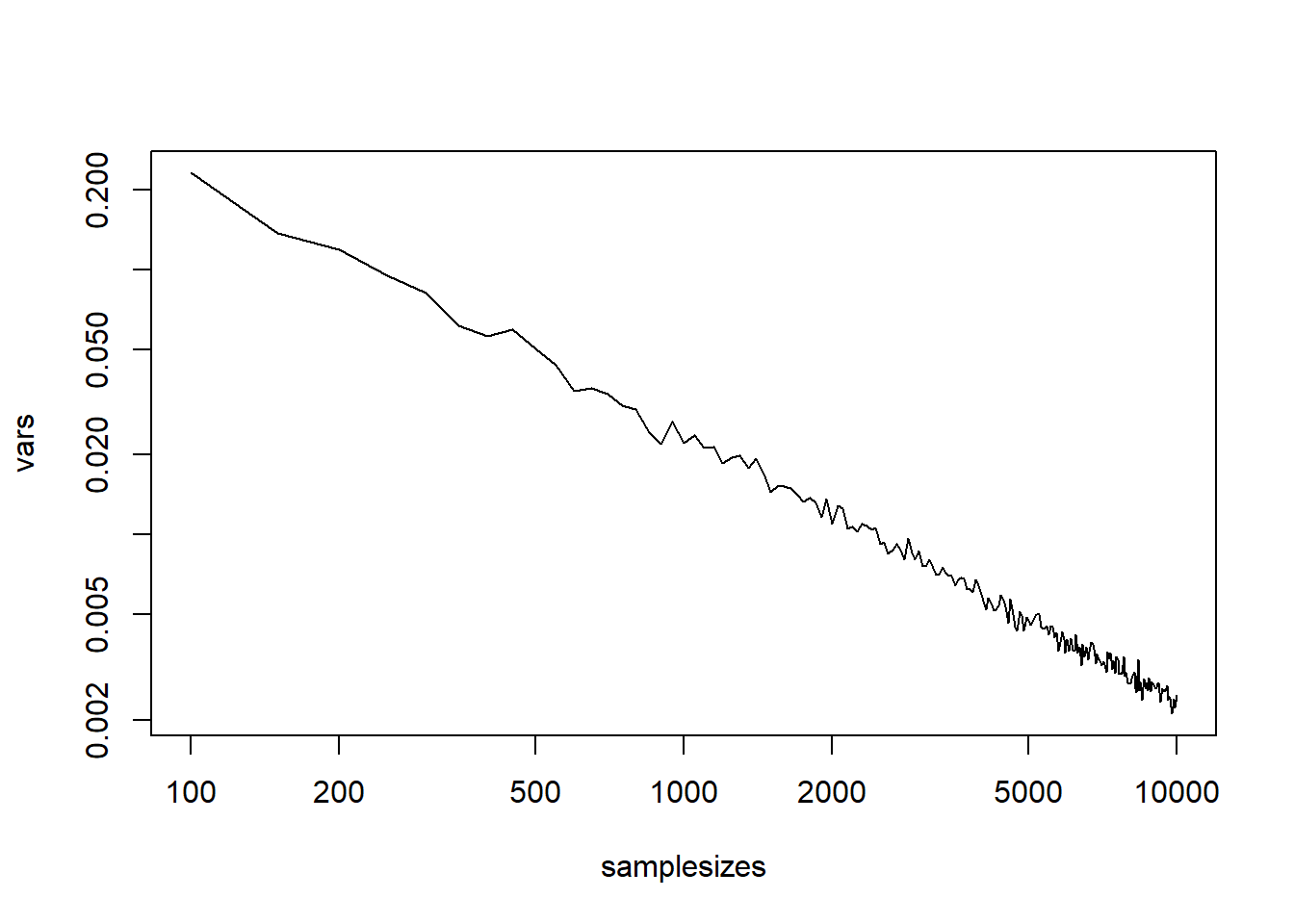# Variance Of Sample Kurtosis

## Kurtosis

First we define the sample kurtosis function, as well as a matrix version.

Source: Wikipedia

kurtosis1 <- function(x) {
xstand <- x - mean(x)
kurt <- (mean((xstand)^4))/((mean((xstand)^2))^2)
return(kurt)
}
kurtosis <- function(X) {   apply(X,2,kurtosis1)   }

## Generate samples

We select the range of sample sizes and generate a matrix of samples arranged in columns, for each size.

samplesizes <- seq(minsize<-100,maxsize<-10000,stepsize<-50)
nsizes <- length(samplesizes)
nsample.persize <- 500
samples <- vector('list',nsizes)
for (i in 1:nsizes) {
samples[[i]] <- matrix(rnorm(samplesizes[i]*nsample.persize),samplesizes[i])
}

We used 500 samples of each size ranging from 100 to 10000 in steps of 50.

## Calculate Kurtosis

We use parallel processing to speed up the computation. Set cluster size to number of available cores.

library(parallel)
cl <- makeCluster(4)
nul <- clusterExport(cl,c('kurtosis1','kurtosis'))
system.time({
outputs <- parLapply(cl,samples,kurtosis)
})
##    user  system elapsed
##    4.83   12.77   37.06
stopCluster(cl)

## Variances

We calculate the variance for each sample size and do a regression on the results.

vars <- unlist(lapply(outputs,var))
lvars <- log(vars); logn <- log(samplesizes)
summary(m1 <- lm(lvars~logn))
##
## Call:
## lm(formula = lvars ~ logn)
##
## Residuals:
##       Min        1Q    Median        3Q       Max
## -0.180953 -0.043097  0.003116  0.044296  0.166688
##
## Coefficients:
##              Estimate Std. Error t value            Pr(>|t|)
## (Intercept)  3.141518   0.042761   73.47 <0.0000000000000002 ***
## logn        -0.996792   0.005152 -193.46 <0.0000000000000002 ***
## ---
## Signif. codes:  0 '***' 0.001 '**' 0.01 '*' 0.05 '.' 0.1 ' ' 1
##
## Residual standard error: 0.06569 on 197 degrees of freedom
## Multiple R-squared:  0.9948, Adjusted R-squared:  0.9947
## F-statistic: 3.743e+04 on 1 and 197 DF,  p-value: < 0.00000000000000022
b0 <- exp(coef(m1))
plot(samplesizes,vars,type='l',log='xy')The regression seems good.

## Conclusion

The final estimated formula is $$v\approx$$ 23.1389604 $$n^{-0.9967917}$$.

Thus it is clear that the formula suggested on Wikipedia is correct.

That is $$v\approx \frac{24n(n-1)^2}{(n-3)(n-2)(n+3)(n+5)}$$.

Statistician